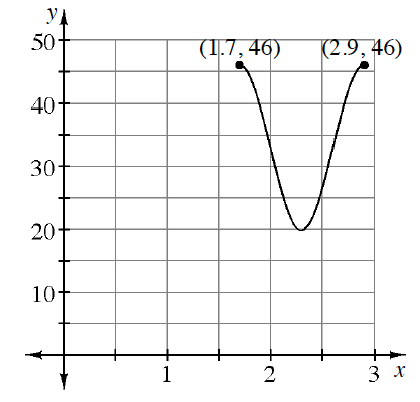### Home > PC3 > Chapter Ch8 > Lesson 8.1.2 > Problem8-34

8-34.

Lauren is playing with a yo-yo and keeping track of its height over time. She has plotted some key points on the graph at right. The $y$‑values are in inches and the $x$‑values are in seconds. Complete the following problems based on the graph.

1. How much time does it take to complete one cycle?

Use the $x$-values to determine this quantity.

2. How long is the string?

This is the difference in the maximum and minimum heights.

3. Write an equation that models the height of the yo-yo over time.

Identify:
1. Amplitude
2. Vertical Shift
3. Horizontal Shift
4. $b$ (frequency)

Note: Part (a) is the period and $b=\frac{2\pi}{p}$
4. Determine the first two times when the yo-yo is exactly $30$ inches above the ground.
Use a graphing calculator to determine where the function crosses $y = 30$.